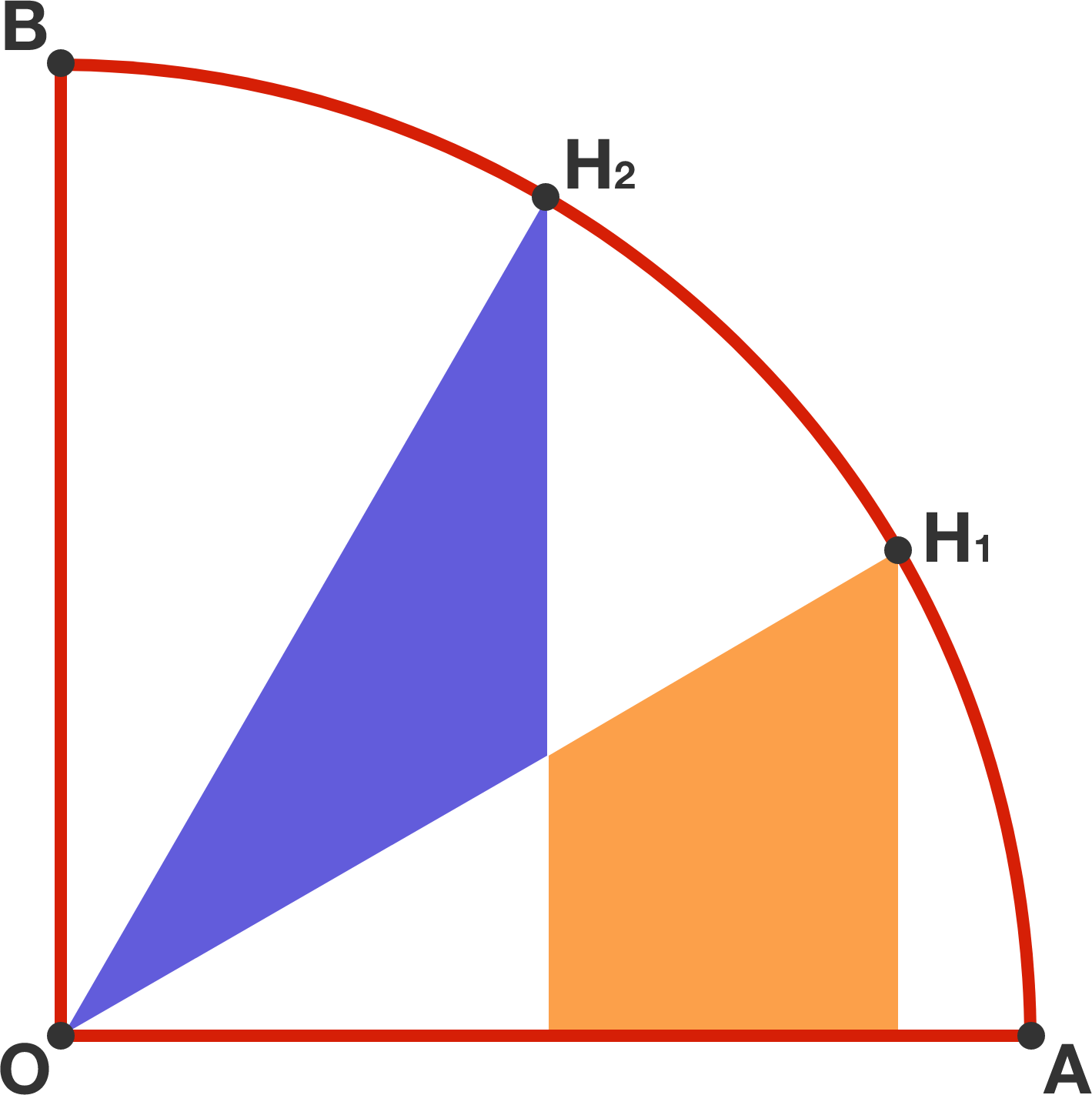# A quarter circle

Geometry Level 1

$OAB$ is a quarter circle, and points $H_1$ and $H_2$ divide the arc into three equal parts. Now, we drop perpendiculars from $H_1$ and $H_2$ to $OA$.Which is larger, the blue triangle or orange quadrilateral?

×